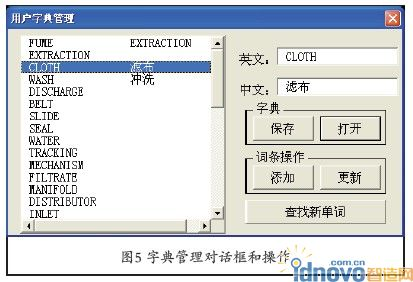(1)图形设置：通过样式管理器、字体设置和标注设置这三种方式对图形定义的字体和标注形式进行管理，以保 证图样能显示中文、字体和标注符合相关标准。

( 2 ) 图形转换：实现对图形中 文字的替换。可选择不同的字典，按 字典中的条目对字字进行“翻译”。

(3)字典管理：用以创建、修改字典。用户创建英汉字典、标准对照、材 料对照字典后，就可通过“图形转换” 操作对图样进行转换。

(4)系统设置：主要方便用户对系统 相关文件的管理。

(2)文本转换模块：主要完成对图形中所有文字的替换，如图4所示。这些文字可能是单行文本、多行文本、属性和标注文字，为了程序的结构清晰，设计Get Text和Set Text两个通用过程处理。 另外，图形中还含有块参照且可能这些块是嵌套的，因此将文本转换设计为一个递归过程。

(3)字典操作：是本程序的重头，鉴于面向对象的优越性，通过构造一 个字典类来实现对数据的维护和操作， 从而把与字典有关的操作全部集中到字典类上。它包含以下内容：常数：

dicmax——定义字典允许的最大的词条数，根据运行效率、内存占用和实际需求设定其数值;属性：Count——字典实际词条数，data——词条;方法：

(4)系统设置：主要指定系统工作路 径、字体文件路径、字典文件路径，并 保存在Windows注册表中。

‘数据

Const dicMAX = 1000 ‘ 定义最大词条数，根据实际需要和系统性能定

Private DicData() As String ‘词条数组

Private dicCount As Integer ‘词条总数

‘只读属性data

Property Get data() As Variant data = DicData

End Property

‘只读属性count

Property Get count() As Integer count = dicCount

End Property

‘A d d操作，成功返回T r u e,e n g— 原文，chn—译文

As String) As Boolean

If dicCount >= dicMAX Then Add = False: Exit Function ‘如果词条数超过 最大值则添加失败

R e D i m P r e s e r v e D i c D a t a ( 1 , dicCount) ‘重定义词条数组大小

DicData(0, dicCount) = eng DicData(1, dicCount) = chn dicCount = dicCount + 1

End Function

‘ Update 操作，成功返回True,eng—原文，chn—译文，Index—索引

Function Update(index As Integer,

eng As St ring, chn As St ring) As

Boolean

If index >= dicMAX Or index < 0

Then Update = False: Exit Function DicData(index, 0) = eng DicData(index, 1) = chn

Update = True

End Function

‘Search操作，成功返回查到的词 条索引，失败返回0

Function Search(eng AsString, ByRef chn As String) As Integer

For i = 0 To dicCount – 1

‘通过一个循环逐条比较

If DicData(i, 0) = eng Then

If DicData(i, 1) = "" Then

Search = 1 ‘ 查到原文，没有对应译文，返回1

Else

Search = 2 ‘ 查到原文且有对应译文，返回2

chn = DicData(i, 1) End If

Exit Function

End If

Next

Search = 0‘没有查到原文，返回0

End Function

‘内部过程MyReplace，是Cov的 主过程，提取出来方便以后修改或升级

Private Function MyReplace(source As String, find As String, replace As String, op As Integer) As String

ls = Len(source)

lf = Len(find)

Dim k As Integer

k = I n S t r ( 1 , s o u r c e , f i n d , vbTextCompare) ‘在原文本中查找要查找的子串

While (k > 0)

Select Case op ‘ 按op的值

Case 1:

MyReplace = Left(source, k - 1) &replace ‘直接替换 Case 2:

MyReplace = Left(source, k - 1) &"(" & replace & ")"言之‘替换并在两边加 注括号

Case 3:

MyReplace = Left(source, k + lf - 1)& "(" & replace & ")" ‘保留原文并加括号 End Select

source = Right(source, ls - k + 1 - lf)

k= In Str(k+lf, source, find, vbTextCompare) 继续查找下一处

Wend

MyReplace = MyReplace & source

End Function

‘Cov操作，s—原文本，op—翻译选 项;返回译文，没有翻译时译文=原文本

Function Cov(s As String, op As

Integer) As String

Cov = s

For i = 0 To dicCount - 1

Cov = MyReplace(Cov, DicData(0, i), DicData(1, i), op)

Next

End Function

Boolean

On Error GoTo l1

Open fn For Input As #1

‘打开文件

Dim e As String, c As String

While Not EOF(1)

Input #1, e, c‘读入词条 Add e, c ‘加入字典 Wend

Close 1 ‘关闭文件 Load = True

Exit Function l1:

End Function

‘S a v e操作，写出字典文件，f n— 字典文件的全路径和名称

Function Save(fn As String) As

Boolean

On Error GoTo l1

Open fn For Output As #1

‘打开文件，准备写入

For i = 0 To dicCount - 1

Write #1, DicData(0, i), DicData(1, i)

‘写词条 Next

Close 1 关闭文件 Save = True

Exit Function l1:

Save = False ‘ 如 遇 错 误，返回失败

End Function

‘Clear操作，清空

Sub Clear()

dicCount = 0

‘词条计数值勤0

ReDim DicData(1, 0)

‘词条数组清空 End Sub

‘ 初 始 操 作 ， 将 dicCount值0，(在VB中 可省略)

Private Sub Class_

Initialize()

dicCount = 0

End Sub◎个人进行C A D二次开发来创建一 个简单实用的图形工具是完全能够实 现的;

◎个人用V B A进行C A D二次开发效率 高，可维护性强，不存在与AutoCAD各版 本不兼容的问题;

◎通过对话框的形式实现软件与用 户的交互很直观，也很方便。